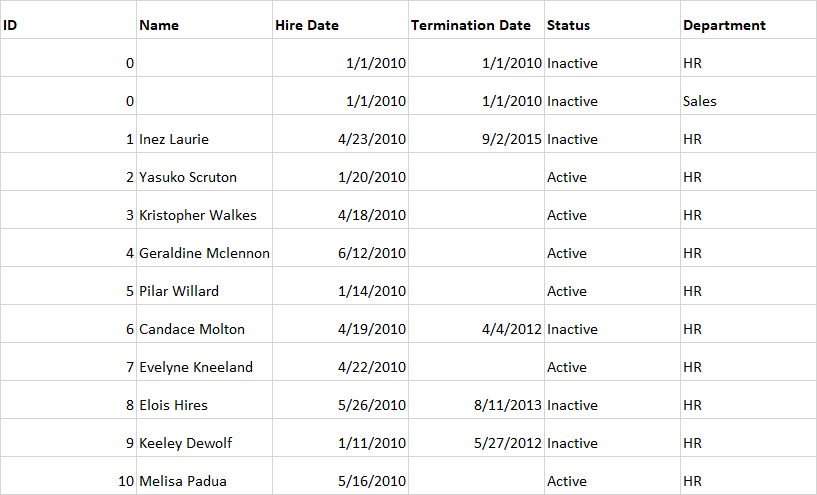cancel
Showing results for
Did you mean:## Kaplan Meier Survival Curves with Power BI - Part 1

Introduction

Inspired by a post on the Power BI Community site, I read through this fine article by Chris Short on LinkedIn Pulse, Survival Curves in Tableau with HR Data. Overall, this technique looked really interesting and I wondered if I could do something similar in Power BI. Turns out that you can and this article will show you how.

I will not reiterate the history of Kaplan Meier Survival Curves. If you just have to know, Chris Short's article referenced above does a great job of summarizing their history and use or you could read this Wikipedia article.

## Building the Curve

Basically, what we want to do is to use some HR data with a hire date and termination date to build a Survival Curve that describes the time between when employees are hired and terminated.

### Step 1: Get the Data

Turns out that the data for this is incredibly simple, essentially a single table. I used the same exact data that Chris Short used in his article. The data can be downloaded here. I simply saved the data to an Excel file. The data looks something like this:### Step 2: Load into Power BI and Create Calculated Fields

This next step starts very similar to the Tableau process, once you have the Excel file, you can load it into Power BI Desktop by choosing Home | Get Data | Excel from the ribbon. Point to Sheet1 and Load the data. Rename the table to "HRData" and then create the following three calculated columns:

```Count = 1
Days = IF([Status]="Active",DATEDIFF(HRData[Hire Date],TODAY(),DAY),DATEDIFF(HRData[Hire Date],HRData[Termination Date],DAY))
Event = IF(HRData[Status] = "Inactive",1,0)```

Count: This is a simple counter column, sure we could use the COUNT or COUNTROWS aggregation instead, but this makes things a little more obvious.

Days: This is the "time to event" calculation. In this case, it is the tenure of the employee in days. Unlike the Tableau method, we do not convert this to years but leave it as days.

Event: As in the Tableau method, we define our event as a person becoming inactive.

Step 3: Do Some Data Modeling

Because Power BI works a little differently than Tableau, we need to do some additional data modeling. The Tableau technique relies on a handy little function called PREVIOUS_VALUE, which is really nifty. But we don't really have an equivalent for PREVIOUS_VALUE when working with Power BI Measures so we will need to do some additional work. The reason we need this is that the Kaplan Meier equation is essentially a running product, which is kind of like a running total except that you are doing multiplication instead of addition.

We start by creating a New Table using the following formula:

`KMDaysAll = GENERATESERIES(0,MAX(HRData[Days]),1)`

This creates a table with a single Value column that goes from 0 to the maximum Days in the HRData table in increments of 1. Rename the Value column to Days. Switch to the Relationships tab and build a relationship between the Days columns in HRData and KMDaysAll.

Now, in KMDaysAll, create the following calculated columns:

```Count = var records = CALCULATE(SUM(HRData[Count]),RELATEDTABLE(HRData)) RETURN IF(ISBLANK(records),0,records)
e_i = var records = CALCULATE(SUM(HRData[Event]),RELATEDTABLE(HRData)) RETURN IF(ISBLANK(records),0,records)
Running = SUMX(FILTER(ALL(KMDaysAll),[Days]<=EARLIER([Days])),KMDaysAll[Count])
d_i = [Count] + CALCULATE(SUM([Count]),ALL(KMDaysAll)) - [Running]
1-e_i/d_i = 1-[e_i]/[d_i]```

OK, these deserve some explanation:

Count: This just gets the number of HRData records that correspond to each day.

e_i: This represents the number of events that occur over time. This is the numerator in our Kaplan Meier's calculation.

Running: This is a running total of the Count of HRData records

d_i: This will be the denominator for the Kaplan Meier calculation, which represents the total population of people since the last time period (ti – 1)

1-e_i/d_i: This is the Kaplan Meier curve calculation that we will need to perform a running product on.

So, we now create a measure for the calculation our Kaplan Meier curve. This is the Survival Curve, or specifically the probability of survival at ti – which is 1 – the hazard function (probability of not surviving). We do this with a measure as follows:

`MyKMDaysAll = CALCULATE(PRODUCT('KMDaysAll'[1-e_i/d_i]), FILTER(ALLSELECTED('KMDaysAll'[Days]),ISONORAFTER('KMDaysAll'[Days], MAX('KMDaysAll'[Days]), DESC)))`

Basically, this is your standard running total calculation when you use a Running Total Quick Measure except that we use PRODUCT as our aggregation. We are using a Running Product essentially in order to get around not having a PREVIOUS_VALUE function.

### Step 4: Build the Curve

Now you can just choose a Line chart visual and put Days from the KMDaysAll table in the x-axis and the MyKMDaysAll measure in the y-axis. A little formatting and you should get something like the image below:Conclusion

This is Part 1. Stay tuned for Part 2 to see how to separate out and view individual Departments!